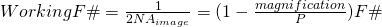# Numerical Aperture

The Numerical Aperture (“NA”) is a measure for the resolution of a lens.

At given working distance the diffraction limited lens like a telecentric lens with the higher Numerical Aperture has the better resolution

The Numerical Aperture is calculated as sine of half the aperture angle multiplied by the refraction index of the media.

According to Snells Law, the Numerical aperture of the lens stays constant across various media :From the NA , you can calulate the F-Number of a lens from the above equition with n about 1 and because (for small angles sin(x) is about tan(x)) to a simplified:If magnification and pupil magnification P are known, we can calculate the effective F-number :and alsoWith the object distance increasing to infinity, the Working F# nears the F#.